# Graphing Linear Inequalities in Two Variables Linear Equations

• Slides: 7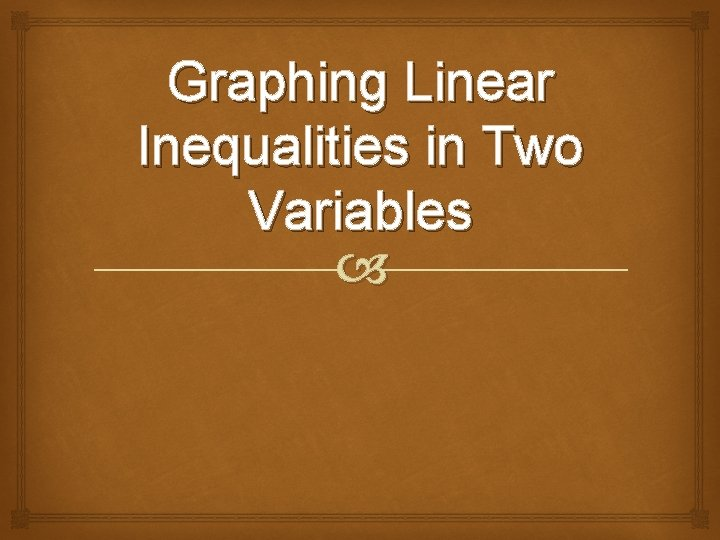Graphing Linear Inequalities in Two VariablesLinear Equations in Two Variables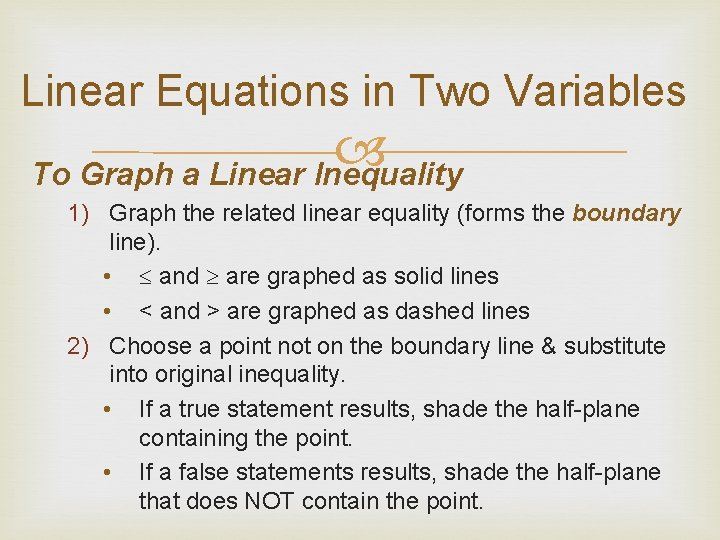Linear Equations in Two Variables To Graph a Linear Inequality 1) Graph the related linear equality (forms the boundary line). • and are graphed as solid lines • < and > are graphed as dashed lines 2) Choose a point not on the boundary line & substitute into original inequality. • If a true statement results, shade the half-plane containing the point. • If a false statements results, shade the half-plane that does NOT contain the point.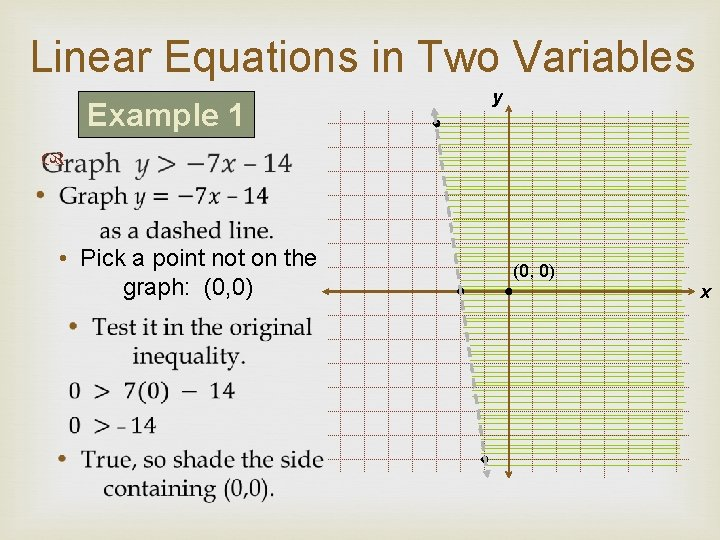Linear Equations in Two Variables Example 1 y • Pick a point not on the graph: (0, 0) xLinear Equations in Two Variables Example Graph 3 x 2+ 5 y – 2 • Graph 3 x + 5 y = – 2 as a solid line. • Pick a point not on the graph: (0, 0), but just barely • Test it in the original inequality. 3(0) + 5(0) – 2 0 – 2 • False, so shade the side that does not contain (0, 0). y (0, 0) x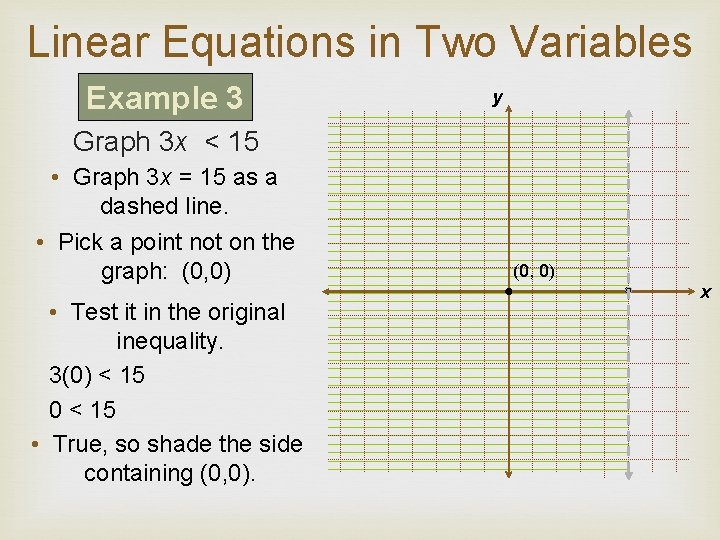Linear Equations in Two Variables Example 3 y Graph 3 x < 15 • Graph 3 x = 15 as a dashed line. • Pick a point not on the graph: (0, 0) • Test it in the original inequality. 3(0) < 15 0 < 15 • True, so shade the side containing (0, 0) x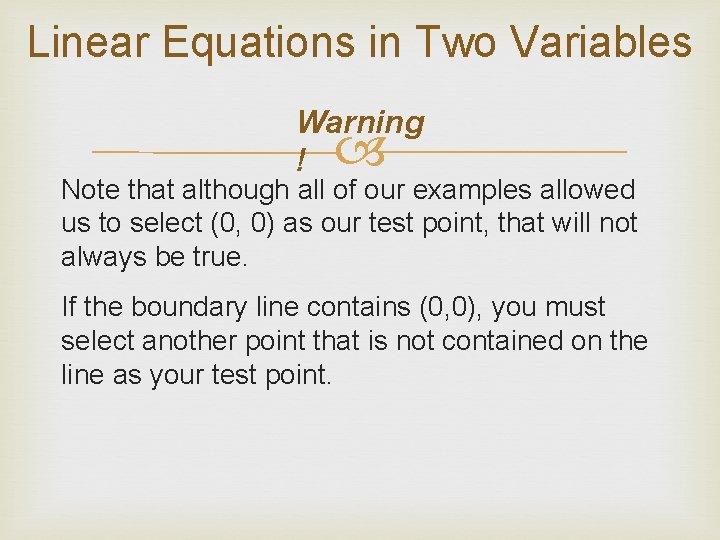Linear Equations in Two Variables Warning ! Note that although all of our examples allowed us to select (0, 0) as our test point, that will not always be true. If the boundary line contains (0, 0), you must select another point that is not contained on the line as your test point.